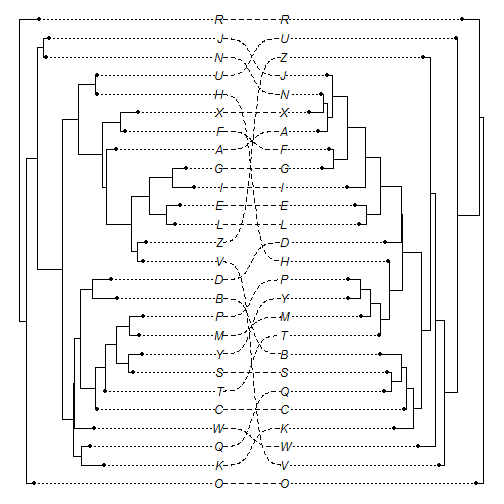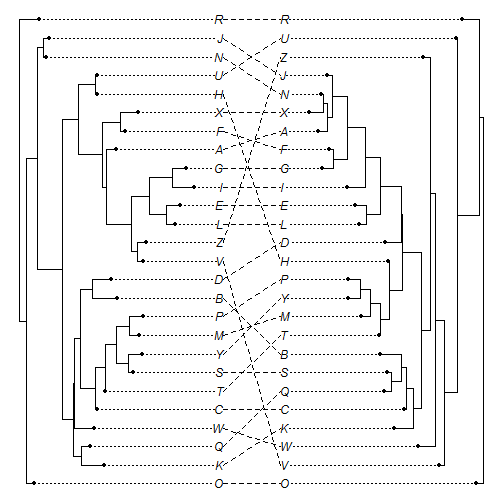## Friday, August 5, 2016

### Plotted `"cophylo"` object with sigmoidal linking lines

A user recently asked if it was possible to plot an object of class `"cophylo"` using curved linking lines. (Actually, he asked for a whole bunch of other features that I haven't gotten to yet.)

I thought this might look cool - so I tried it using a function modified from this stackoverflow example.

This is what it looks like. Here I am not using any association table, just trying to match the tip labels of the two trees exactly:

``````library(phytools)
t1
``````
``````##
## Phylogenetic tree with 26 tips and 25 internal nodes.
##
## Tip labels:
##  R, O, Q, K, C, Y, ...
##
## Rooted; includes branch lengths.
``````
``````t2
``````
``````##
## Phylogenetic tree with 26 tips and 25 internal nodes.
##
## Tip labels:
##  R, O, Q, K, C, Y, ...
##
## Rooted; includes branch lengths.
``````
``````obj<-cophylo(t1,t2)
``````
``````## Rotating nodes to optimize matching...
## Done.
``````
``````plot(obj,link.type="curved")
``````Compared to the “classic” format:

``````plot(obj)
``````The updated code can be viewed here, and installed from GitHub using devtools as normal.

The trees were simulated using phytools and phangorn as follows:

``````library(phangorn)
t1<-rtree(n=26,tip.label=LETTERS)
t2<-rNNI(t1,moves=100)
``````